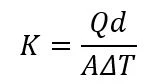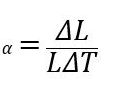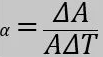•收藏本站
•在线留言
•网站地图# 资讯中心K = 热导率 (W/(mK)) 或 (Btu/(hrft*°F))

Q = 传热量 (W) 或 (Btu)

d = 两个等温面之间的距离 (m) 或 (ft)

A = 表面积 (m²) 或 (ft²)

ΔT = 温度变化 (K) 或 (°F)

 材料 导热系数 (W/mK) 水 0.598 标准模量 (34 Msi) 碳纤维（仅纤维） 9 到 11 AISI 1018 碳钢 51.9 6061-T6铝 167 超高模量 (110 Msi) 碳纤维（仅纤维） 200 金子 310

- 体积

- 区域

- 线性α = 面积热膨胀系数（K -1或 1/K）或（°F -1或 1/°F）

L = 原始长度 (m) 或 (ft)

ΔL = 长度变化 (m) 或 (ft)

ΔT = 温度变化 (K) 或 (°F)α = 面积热膨胀系数（K -1或 1/K）或（°F -1或 1/°F）

A= 原始面积 (m²) 或 (ft²)

Delta A= 面积变化 (m²) 或 (ft²)

Delta T = 温度变化 (K) 或 (°F)

 材料 热膨胀系数 (K -1 ) 钻石 0.1 × 10 -5 碳纤维层压板（0/90 编织） 0.15 × 10 -5 AISI 1018 碳钢 1.2 x 10 -5 金子 1.42 × 10 -5 6061-T6铝 2.36 × 10 -5 带领 2.9 × 10 -5

?
?
?上一篇：粘合碳纤维条的技巧和窍门
?下一篇：碳纤维管的用途